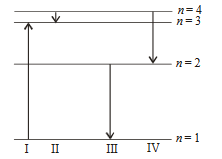## Filters

Sort by :
Clear All
Q
Engineering
113 Views   |

If radius of the nucleus is estimated to be 3.6 fermi then the radius of nucleus be nearly

• Option 1)

• Option 2)

• Option 3)

• Option 4)

As we learnt in Binding energy per nucleon -   - wherein This graph shows the stability of nuclei many nuclear phenomena can be explained by this graph     Correct option is 3. Option 1) This is an incorrect option. Option 2) This is an incorrect option. Option 3) This is the correct option. Option 4) This is an incorrect option.
Engineering
132 Views   |

Starting with a sample of pure , 7/8 of it decays into Zn in 15 min . The corresponding half-­life is

• Option 1)

• Option 2)

• Option 3)

• Option 4)

As we learnt in Number of nuclei after disintegration -  or  - wherein Number of nucleor activity at a time is exponentional function     Hence   t = 3 T1/2 given t = 15 min. Correct option is 1. Option 1) This is the correct option. Option 2) This is an inocrrect option. Option 3) This is an inocrrect option. Option 4) This is an inocrrect option.
Engineering
971 Views   |

The diagram shows the energy levels for an electron in a certain atom. Which transition shown represents the emission of a photon with the most energy?• Option 1)

• Option 2)

• Option 3)

• Option 4)

As we learnt in Energy emitted due to transition of electron - - wherein    Highest difference of energy is between n = 1 and n = 3.  According to . Correct option is III. Since I is absorption of photon. Correct option is 3. Option 1) This is an incorrect option. Option 2) This is an incorrect option. Option 3) This is the correct option. Option 4) This is an incorrect option.
Exams
Articles
Questions